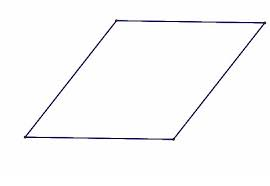## How to Calculate and Solve for the Length and Perimeter of a Rhombus | Nickzom CalculatorThe image above is a rhombus.

To compute the area of a rhombus, one essential parameter is needed and this parameter is length of side (a).

The formula for calculating the perimeter of a rhombus:

P = 4a

Where;
P = Perimeter of the rhombus
a = length of side

Let’s solve an example;
Find the perimeter of a rhombus when the length of side is 30 cm.

This implies that;
a = Length of side = 30 cm

P = 4a
P = 4 x 30
P = 120

Therefore, the perimeter of the rhombus is 120 cm.

Calculating the Length of Side using the Perimeter of the Rhombus.

a = P / 4

Where;
a = Length of Side
P = Perimeter of the rhombus

Let’s solve an example;
Given that the perimeter of the rhombus is 180 cm. Find the length of side?

a = P / 4

This implies that;
P = perimeter of the rhombus = 180 cm

a = P / 4
a = 180 / 4
a = 45

Therefore, the length of side is 45 cm.

## How to Calculate and Solve for the Length of the Diagonal and Area of a Rhombus | The Calculator Encyclopedia

The above image is a rhombus.

To compute the area of a rhombus, two essential parameters are needed and this parameters are length of the diagonal (p) and length of the diagonal (q).

The formula for calculating the area of a rhombus:

A = pq ⁄ 2

Where;
A = Area of the rhombus
p = length of the diagonal
q = length of the diagonal

Let’s solve an example;
Find the area of a rhombus when the length of the diagonal (p) is 10 cm and the length of the diagonal (q) is 18 cm.

This implies that;
p = length of the diagonal = 10 cm
q = length of the diagonal = 18 cm

A = pq2
A = 10 x 18 / 2
A = 180 / 2
A = 90

Therefore, the area of the rhombus is 90 cm2.

Calculating the length of the diagonal (p) using Area of the Rhombus and length of the diagonal (q).

p = 2A / q

Where;
A = Area of the rhombus
q = length of the diagonal

Let’s solve an example;
Given that the length of the diagonal (q) is 20 cm with an area of 60 cm2. Find the length of the diagonal (p)?

This implies that;
A = Area of the rhombus = 60 cm2
q = length of the diagonal = 20 cm

p = 2A / q
p = 2 x 60 / 20
p = 120 / 20
p = 6

Therefore, the length of the diagonal is 6 cm.